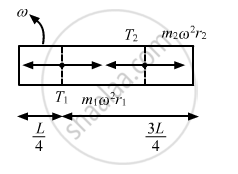Share

# A Train a Runs from East to West and Another Train B of Same Mass Runs from West to East at Same Speed Along the Equator. a Presses the Track with a Force F1 and B Presses the Track with a Force F2. - Physics

ConceptCircular Motion

#### Question

A train A runs from east to west and another train B of the same mass runs from west to east at the same speed along the equator. A presses the track with a force F1 and B presses the track with a force F2

• F1 > F2.

• F1 < F2

• F1 = F2

• the information is insufficient to find the relation between F1 and F2

#### Solution

F1 > F

When the trains are moving, effective angular velocity of both the trains are different (as shown in the figure).Effective angular velocity of train B is more than that of train A.
Normal force with which both the trains push the tracks is given as :  $\text{ N = mg - mR} \omega '^2$  From the above equation, we can conclude that F1 > F2.

Is there an error in this question or solution?

#### APPEARS IN

Solution A Train a Runs from East to West and Another Train B of Same Mass Runs from West to East at Same Speed Along the Equator. a Presses the Track with a Force F1 and B Presses the Track with a Force F2. Concept: Circular Motion.
S